# SAS - Operators

An operator in SAS is a symbol which is used in a mathematical, logical or comparison expression. These symbols are in-built into the SAS language and many operators can be combined in a single expression to give a final output.

Below is a list of SAS category of operators.

• Arithmetic Operators
• Logical Operators
• Comparison Operators
• Minimum/Maximum Operators
• Concatenation Operator

We will look at each of the one by one. The operators are always used with variables that are part of the data that is being analyzed by the SAS program.

## Arithmetic Operators

The below table describes the details of the arithmetic operators. Let’s assume two data variables V1 and V2with values 8 and 4 respectively.

Operator Description Example
- Subtraction V1-V2=4
* Multiplication V1*V2=32
/ Division V1/V2=2
** Exponentiation V1**V2=4096

### Example

```DATA MYDATA1;
input @1 COL1 4.2	@7 COL2 3.1;
Sub_result = COL1-COL2;
Mult_result = COL1*COL2;
Div_result = COL1/COL2;
Expo_result = COL1**COL2;
datalines;
11.21 5.3
3.11  11
;
PROC PRINT DATA = MYDATA1;
RUN;
```

On running the above code, we get the following output.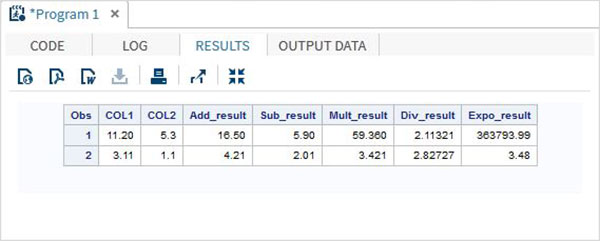## Logical Operators

The below table describes the details of the logical operators. These operators evaluate the Truth value of an expression. So the result of logical operators is always a 1 or a 0. Let’s assume two data variables V1 and V2with values 8 and 4 respectively.

Operator Description Example
& The AND Operator. If both data values evaluate to true then the result is 1 else it is 0. (V1>2 & V2 > 3) gives 0.
| The OR Operator. If any one of the data values evaluate to true then the result is 1 else it is 0. (V1>9 & V2 > 3) is 1.
~ The NOT Operator. The result of NOT operator in form of an expression whose value is FALSE or a missing value is 1 else it is 0. NOT(V1 > 3) is 1.

### Example

```DATA MYDATA1;
input @1 COL1 5.2	@7 COL2 4.1;
and_=(COL1 > 10 & COL2 > 5 );
or_ = (COL1 > 12 | COL2 > 15 );
not_ = ~( COL2 > 7 );
datalines;
11.21 5.3
3.11  11.4
;
PROC PRINT DATA = MYDATA1;
RUN;
```

On running the above code, we get the following output.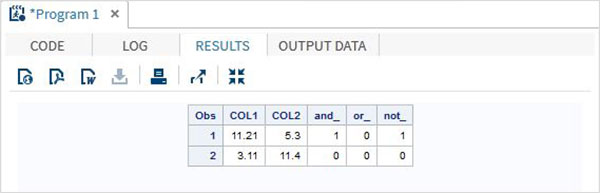## Comparison Operators

The below table describes the details of the comparison operators. These operators compare the values of the variables and the result is a truth value presented by 1 for TRUE and 0 for False. Let’s assume two data variables V1 and V2with values 8 and 4 respectively.

Operator Description Example
= The EQUAL Operator. If both data values are equal then the result is 1 else it is 0. (V1 = 8) gives 1.
^= The NOT EQUAL Operator. If both data values are unequal then the result is 1 else it is 0. (V1 ^= V2) gives 1.
< The LESS THAN Operator. (V2 < V2) gives 1.
<= The LESS THAN or EQUAL TO Operator. (V2 <= 4) gives 1.
> The GREATER THAN Operator. (V2 > V1) gives 1.
>= The GREATER THAN or EQUAL TO Operator. (V2 >= V1) gives 0.
IN The IN Operator. If the value of the variable is equal to any one of the values in a given list of values, then it returns 1 else it returns 0. V1 in (5,7,9,8) gives 1.

### Example

```DATA MYDATA1;
input @1 COL1 5.2	@7 COL2 4.1;
EQ_ = (COL1 = 11.21);
NEQ_= (COL1 ^= 11.21);
GT_ = (COL2 => 8);
LT_ = (COL2 <= 12);
IN_ = COL2 in( 6.2,5.3,12 );
datalines;
11.21 5.3
3.11  11.4
;
PROC PRINT DATA = MYDATA1;
RUN;
```

On running the above code, we get the following output.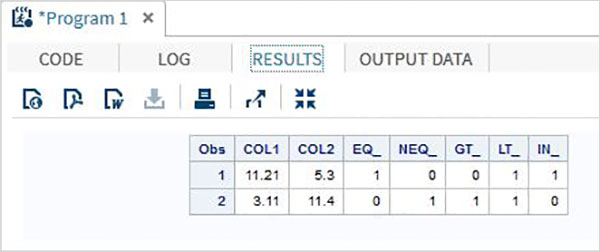## Minimum/Maximum Operators

The below table describes the details of the Minimum/Maximum operators. These operators compare the values of the variables across a row and the minimum or maximum value from the list of values in the rows is returned.

Operator Description Example
MIN The MIN Operator. It returns the minimum value form the list of values in the row. MIN(45.2,11.6,15.41) gives 11.6
MAX The MAX Operator. It returns the maximum value form the list of values in the row. MAX(45.2,11.6,15.41) gives 45.2

### Example

```DATA MYDATA1;
input @1 COL1 5.2	@7 COL2 4.1 @12 COL3 6.3;
min_ = MIN(COL1 , COL2 , COL3);
max_ = MAX( COL1, COl2 , COL3);
datalines;
11.21 5.3 29.012
3.11  11.4 18.512
;
PROC PRINT DATA = MYDATA1;
RUN;
```

On running the above code, we get the following output.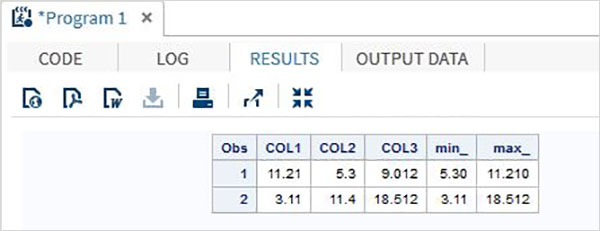## Concatenation Operator

The below table describes the details of the Concatenation operator. This operator concatenates two or more string values. A single character value is returned.

Operator Description Example
|| The concatenate Operator. It returns the concatenation of two or more values. 'Hello'||' World' gives Hello World

### Example

```DATA MYDATA1;
input  COL1 \$	COL2 \$  COL3 \$;
concat_ = (COL1 || COL2 || COL3);
datalines;
Tutorial s point
simple easy learning
;
PROC PRINT DATA = MYDATA1;
RUN;
```

On running the above code, we get the following output.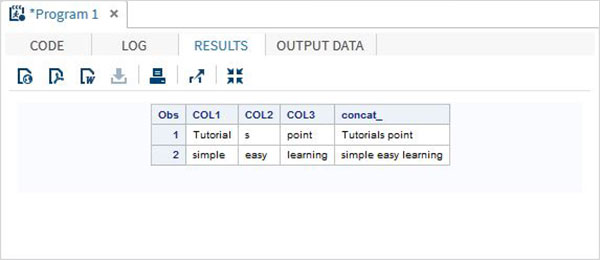## Operators Precedence

The operator precedence indicates the order of evaluation of the multiple operators present in complex expression. The below table describes the order of precedence with in a group of operators.

Group Order Symbols
Group I Right to Left ** + - NOT MIN MAX
Group II Left to Right * /
Group III Left to Right + -
Group IV Left to Right ||
Group V Left to Right < <= = >= >